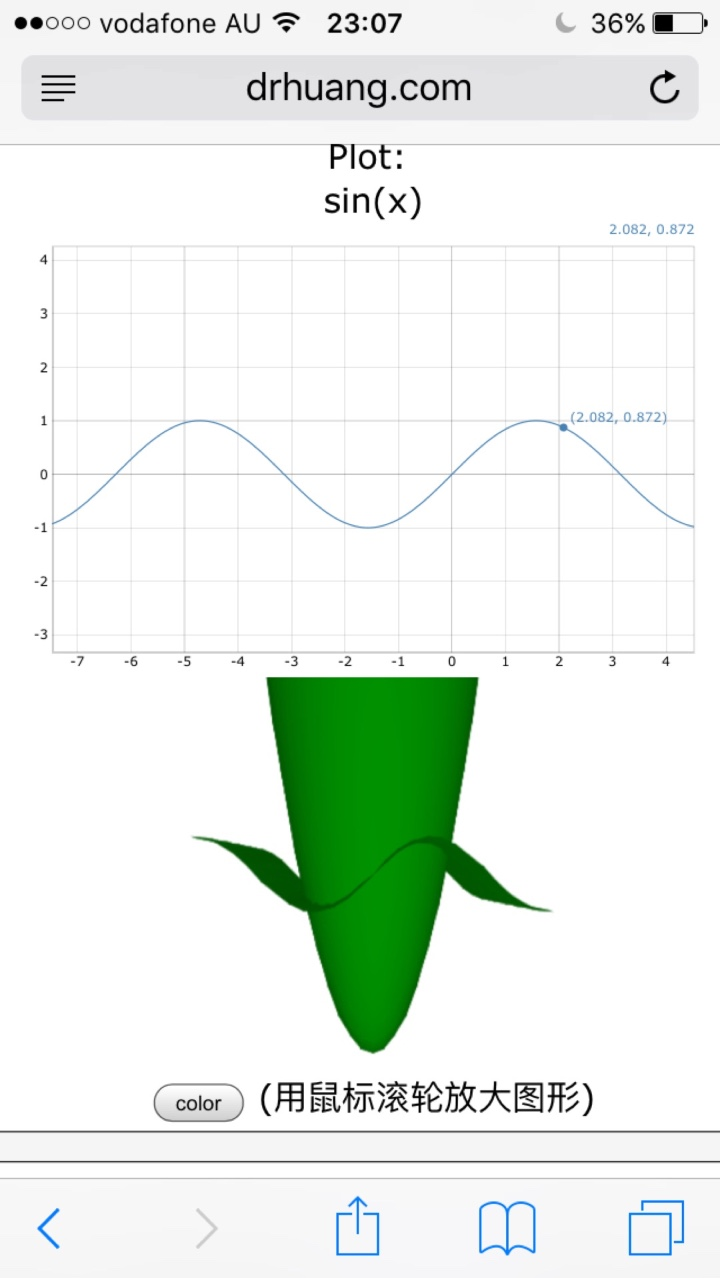﻿﻿ Fractional Calculus Computer Algebra System, Electrochemistry Software, Massage+++=﻿

# Surface in 3D

You may find here some examples of algebraic surfaces plotted with implicit3D and plot3D.

If you click on a small image, you will have the menu of the plotter with the equation of the surfaces already loaded. Which will allow you to plot a bigger image of the surface, while playing with the parameters (colors, viewing angles, zoom, spin, etc).

DemoEquationDescriptionx2+y2-z2=0 Quadratic cone.x2+y2+z2+2x y z=1 Cubic with 4 singular points.x4+y4+z4+5x y z=0 A quartic surface with 4 branches touching at the origine.x3+y3+z3=(x+y+z) a cubic surface with rotation.x2 y2+y2 z2+z2 x2=(x2+y2+z2-0.75) a quartic surface.

## Reference 参阅

• example 例题
• function graph 制图
• graphics 制图法
• animation 动画 ﻿